Optimizing Marketing Investment

Table Functions of our .NET libraries finaquant® protos (non-commercial) or finaquant® calcs (commercial) can be used to formulate evaluation function (also known as objective or fitness function) and constraints in any optimization scenario quite easily without cumbersome database programming; following model example shows how.

The output of an evaluation function can be measures like profit, time or costs, depending on the figure you want to minimize or maximize. In the following example, we want to get optimal marketing investment amounts per brand (value drivers) in order to maximize total profit from sales.

We will use Nelder-Mead Solver from Microsoft Solver Foundation (free .NET library) to find optimal investment amounts in this example.

You may download the Visual Studio project file with all the C# code required to run this example here.

An online digital products dealer wants to increase its sales through advertisements on internet. Each ad will promote a certain brand (i.e. ads at brand level). The increase in sales for each product depends on (1) investment amount, and (2) sensitivity α of the product.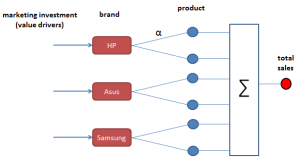Formulas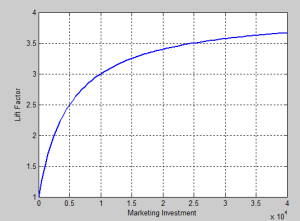Goal: Finding optimal marketing investment amount per brand to maximize profit

Input Tables

There are two input tables:

1. Product table with key figures like price, cost, sensitivity and quantity (normal expected quantity without any advertisement)
2. Marketing Investment table with initial (sub-optimal) investment amounts.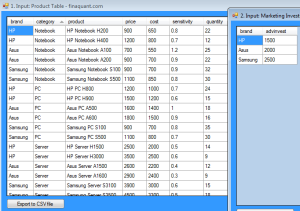Following C# code shows how input tables are created:
Create Input Tables

Formulating Profit with Table Functions

Following C# code shows how profit can be formulated with table functions.

Note: Row Transformer (MatrixTable.TransformRowsDic) is used to apply user-defined formulas on each row of extended product table.
Calculate Profit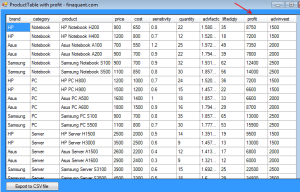Finding Optimal Investment Amounts with a Constraint

Following C# code shows how optimal investment amount for brand each can be obtained with the Nelder-Mead Solver for non-linear optimization models without constraints.

In the code below, objProfit2() is the evaluation function.
Constraint: Total Marketing Investment <= \$30000 Note: Nelder-Mead Solver can solve optimization models without constraints, but there is a way to outtrick this solver: Adding a high penalty to evaluation function if constraints are not satisfied. In this example, this penalty is formulated as follows:

 double ConstraintPenalty = (Math.Sign(totInvest - MaxTotalInvestment) + 1) * 500000;
Find Optimal Investment Amounts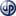Copyright secured by Digiprove © 2014 Tunc Ali Kütükcüoglu